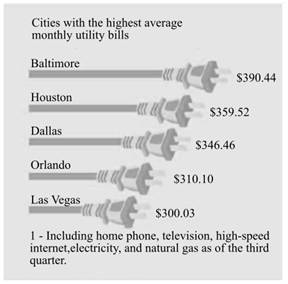Chapter 6.II, Problem 36RE### Contemporary Mathematics for Busin...

8th Edition
Robert Brechner + 1 other
ISBN: 9781305585447

#### Solutions

Chapter
Section### Contemporary Mathematics for Busin...

8th Edition
Robert Brechner + 1 other
ISBN: 9781305585447
Textbook Problem

# Solve the following word problems for the portion, rate, or base.As part of a report you are writing that compares living expenses in various cities, use the chart "Cities with the highest average monthly utility bills” to calculate the following:a. What percent is the Baltimore utility bill of the Las Vegas bill? Round to the nearest whole percent.b. What percent is the Orlando utility bill of the Dallas bill? Round to the nearest tenth of a percent.(a)

To determine

To calculate: The percent of the Baltimore utility bill to the Las Vegas bill and round it to the nearest whole percent, according to a report which compares living expenses in various cities by using the chart,Explanation

Given Information:

Provided chart is,

Formula used:

Step1: Recognize the two known values and the unknown value.

Step2: Pick the formula Rate=PortionBase that solves the unknown value.

Step3: Solve the equation by putting the known values for the letters in the formula Rate=PortionBase.

Calculation:

Provided value of Baltimore is $390.44 and the value of Las Vegas is$300.03.

Hence, the value of Portion is $390.44 and value of base is$300.03.

Here, missing value is percent or rate.

The formula to compute the rate is Rate=PortionBase ……

(b)

To determine

To calculate: The percent of the Orlando utility bill to the Dallas bill (round to the nearest whole percent), according to a report that compares living expenses in various cities by using the chart,### Still sussing out bartleby?

Check out a sample textbook solution.

See a sample solution

#### The Solution to Your Study Problems

Bartleby provides explanations to thousands of textbook problems written by our experts, many with advanced degrees!

Get Started

#### Find more solutions based on key concepts